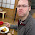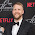## Friday, November 2, 2012

### Easy Command Line Math for Mac and Linux

I found myself going to google frequently for basic math that my feeble brain couldn't handle.
Here's two ways to do it easily on the command line on mac osx and linux variants.
Using echo:
``````\$ echo \$(( 6 / 2 ))
3

\$ echo \$(( 6 * 2 ))
12

\$ echo \$(( 6 / 7 ))
0``````
exp -- evaluate expression
``````expr 300 + 9230
9530

\$ expr 6 \* 32
192

\$ expr 32 \/ 4
8

\$ expr 5 + 5.5
expr: non-numeric argument

\$ expr 6 \/ 7
0``````
I found myself calculating epoch timestamps for 5 or 10 minutes in the future. I typically used this as an expiration in some api method. We can use our new tool to calculate this easily:
``\$ echo \$(( `date +"%s"` + 300 )) # Five minutes in the future``
You can wrap this up in a bash function for easy use later. In my bashrc I have the following function:
``````timestamp(){
echo \$(( `date +"%s"` + \$1 ))
}``````
Which allows me to call it directly from the command line when I need a new expiration:
``````\$ timestamp 300
1351897187``````
Now we can put off learning basic math solving skills for a little while longer.

### Floats

Thanks Bill in the comments for showing how to calculate floats using bc:
``````\$ echo "scale=3; 6/7" | bc
.857``````

1.I find the python interpreter is pretty useful for this sort of stuff too. Does floats as well :). I'll probably being stealing your timestamp function for some scripts.

2.Hah, steal away. I actually use ipython frequently too.

Lately I've really enjoyed going to wolfram alpha for certain types of questions, like "solve for a if 26a + 39b = 8920". It actually doesn't tell you the answer, but solves it with you and reminds you how to do simple algebra :) There's even an api for it.

3.In an instance where a decimal answer is needed, it's very easy to simply pipe to bc. We can use the scale attribute to control how many digits after the decimal point. Using one of your examples:

\$ echo "scale=3; 6/7" | bc
.857

1.Nifty! Thanks for the pro-tip.